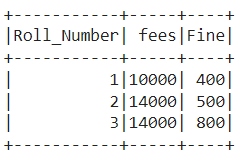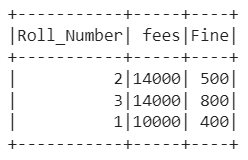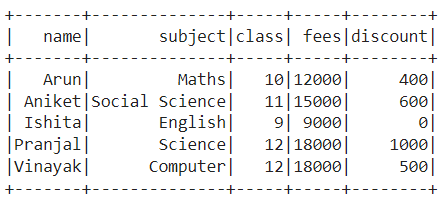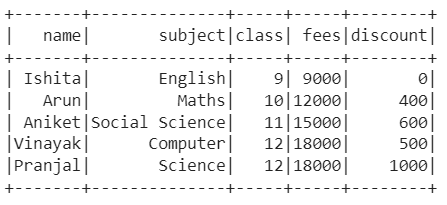GeeksforGeeks App
Open AppBrowser
Continue

# PySpark RDD – Sort by Multiple Columns

In this article, we are going to learn sorting Pyspark RDD by multiple columns in Python.

There occurs various situations in being a data scientist when you get unsorted data and there is not only one column unsorted but multiple columns are unsorted. This situation can be overcome by sorting the data set through multiple columns in Pyspark RDD. You can do it in two ways, either by sorting through the sort() function or by sorting through the orderBy() function. We have explained both ways in this article.

## Methods to sort Pyspark RDD by multiple columns

### Method 1: Sort Pyspark RDD by multiple columns using sort() function

The function which has the ability to sort one or more than one column either in ascending order or descending order is known as the sort() function. The columns are sorted in ascending order, by default. In this method, we will see how we can sort various columns of Pyspark RDD using the sort() function. What we will do is sort the columns in ascending or descending using asc or desc functions respectively.

Syntax: sort(desc(“column_name_1”), asc(“column_name_2”)).show()

Here,

• column_name_1, column_name_2: These are the columns according to which sorting has to be done.

Stepwise Implementation:

Step 1: First of all, import the libraries SparkSession, col, desc, and asc. The SparkSession library is used to create the session. The desc and asc libraries are used to arrange the data set in descending and ascending orders respectively.

```from pyspark.sql import SparkSession
from pyspark.sql.functions import desc, asc```

Step 2: Create a spark session using the getOrCreate() function

`spark_session = SparkSession.builder.getOrCreate()`

Step 3: Then, either create a data set in RDD using parallelize() function or read the CSV file using read.csv function.

`rdd = sc.parallelize([(column_1_data), (column_2_data), (column_3_data)])`

or

```csv_file = spark_session.read.csv('#Path of CSV file',
sep = ',', inferSchema = True, header = True)
rdd = csv_file.rdd```

Step 4: Convert the RDD data set to the Pyspark data frame using the toDF() function

```columns = ["column_name_1","column_name_2","column_name_3"]
data_frame = rdd.toDF(columns)```

or

`data_frame = rdd.toDF()`

Step 5: Finally, sort the data set in either ascending order or descending order using sort, asc, and desc functions respectively.

`data_frame.sort(desc("column_name_1"), asc("column_name_2")).show()`

Example 1:

In this example, we have created the RDD data set and converted it to a Pyspark data frame with columns ‘Roll_Number‘, ‘fees‘, and ‘Fine‘ as given below. Then, we sorted the data set using the sort() function through the column fees in descending order and column fine in ascending order.## Python3

 `# Pyspark RDD program to sort by multiple columns` `# Import the libraries SparkSession, desc and asc``from` `pyspark.sql ``import` `SparkSession``from` `pyspark.sql.functions ``import` `desc, asc` `# Create a spark session using getOrCreate() function``spark_session ``=` `SparkSession.builder.getOrCreate()` `# Create a sparkContext``sc``=``spark_session.sparkContext` `# Declare the data set in RDD``rdd ``=` `sc.parallelize([(``1``, ``10000``, ``400``),``                      ``(``2``, ``14000` `, ``500``),``                      ``(``3``, ``14000` `, ``800``)])` `# Define the column names to be allotted to the data set``student_columns ``=` `[``"Roll_Number"``,``"fees"``,``"Fine"``]` `# Convert the RDD data set to data frame``data_frame ``=` `rdd.toDF(student_columns)` `# Sort the fees column in ascending order and fine``# column in descending order using sort function``data_frame.sort(desc(``"fees"``), asc(``"Fine"``)).show()`

Output:Example 2:

In this example, we have read the CSV file (link), i.e., 5×5 data set, in RDD format and converted it to Pyspark data frame as given below. Then, we sorted the data set through fees and class in ascending order and names in descending order using the sort() function.## Python3

 `# Pyspark RDD program to sort by multiple columns` `# Import the libraries SparkSession, desc and asc``from` `pyspark.sql ``import` `SparkSession``from` `pyspark.sql.functions ``import` `desc, asc` `# Create a spark session using getOrCreate() function``spark_session ``=` `SparkSession.builder.getOrCreate()` `# Read the CSV file``csv_file ``=` `spark_session.read.csv(``    ``'/content/class_data.csv'``,``    ``sep``=``','``, inferSchema``=``True``, header``=``True``)` `# Declare the data set in RDD``rdd ``=` `csv_file.rdd` `# Convert the RDD data set to data frame``data_frame ``=` `rdd.toDF()` `# Sort the fees and class columns in ascending order and``# name column in descending order using sort function``data_frame.sort(asc(``"fees"``),``                ``asc(``"class"``),``                ``desc(``"name"``)).show()`

Output:### Method 2: Sort Pyspark RDD by multiple columns using orderBy() function

The function which returns a completely new data frame sorted by the specified columns either in ascending or descending order is known as the orderBy() function. In this method, we will see how we can sort various columns of Pyspark RDD using the sort function. What we will do is extract the column using the col function and then sort in ascending or descending using asc or desc functions respectively.

Syntax: orderBy(col(“column_name_1”).desc(), col(“column_name_2”).asc()).show()

Here,

• column_name_1, column_name_2: These are the columns according to which sorting has to be done.

Stepwise Implementation:

Step 1: First of all, import the libraries SparkSession, col, desc, and asc. The SparkSession library is used to create the session. The desc and asc libraries are used to arrange the data set in descending and ascending orders respectively.

```from pyspark.sql import SparkSession
from pyspark.sql.functions import desc, asc```

Step 2: Now, create a spark session using the getOrCreate function.

`spark_session = SparkSession.builder.getOrCreate()`

Step 3: Then, either create a data set in RDD using parallelize function or read the CSV file using read.csv function.

`rdd = sc.parallelize([(column_1_data), (column_2_data), (column_3_data)])`

or

```csv_file = spark_session.read.csv('#Path of CSV file',
sep = ',', inferSchema = True, header = True)
rdd = csv_file.rdd```

Step 4: Convert the RDD data set to the Pyspark data frame using the toDF function.

```columns = ["column_name_1","column_name_2","column_name_3"]
data_frame = rdd.toDF(columns)```

or

`data_frame = rdd.toDF()`

Step 5: Finally, sort the data set in either ascending order or descending order using orderBy(), asc, and desc functions respectively.

`data_frame.orderBy(col("column_name_1").desc(), col("column_name_2").asc()).show()`

Example 1:

In this example, we have created the RDD data set and converted it to a Pyspark data frame with columns ‘Roll_Number‘, ‘fees‘, and ‘Fine‘ as given below. Then, we sorted the data set using the orderBy function through fees in descending order and fine in ascending order.## Python3

 `# Pyspark RDD program to sort by multiple columns` `# Import the libraries SparkSession, desc and asc``from` `pyspark.sql ``import` `SparkSession``from` `pyspark.sql.functions ``import` `desc,``                                  ``col, asc` `# Create a spark session using getOrCreate() function``spark_session ``=` `SparkSession.builder.getOrCreate()` `# Create a sparkContext``sc``=``spark_session.sparkContext` `# Declare the data set in RDD``rdd ``=` `sc.parallelize([(``1``, ``10000``, ``400``),``                      ``(``2``, ``14000` `, ``500``),``                      ``(``3``, ``14000` `, ``800``)])` `# Define the column names to be allotted to the data set``student_columns ``=` `[``"Roll_Number"``,``"fees"``,``"Fine"``]` `# Convert the RDD data set to data frame``data_frame ``=` `rdd.toDF(student_columns)` `# Sort the fees column in ascending order and fine``# column in descending order using orderBy function``data_frame.orderBy(col(``"fees"``).desc(),``                   ``col(``"Fine"``).asc()).show()`

Output:Example 2:

In this example, we have read the CSV file (link), in RDD format and converted it to Pyspark data frame as given below. Then, we sorted the data set using the orderBy() function through column fees and column class in ascending order as well as names in ascending order.## Python3

 `# Pyspark RDD program to sort by multiple columns` `# Import the libraries SparkSession, desc, col and asc``from` `pyspark.sql ``import` `SparkSession``from` `pyspark.sql.functions ``import` `desc, col, asc` `# Create a spark session using getOrCreate() function``spark_session ``=` `SparkSession.builder.getOrCreate()` `# Read the CSV file``csv_file ``=` `spark_session.read.csv(``'/content/class_data.csv'``,``                                  ``sep ``=` `','``,``                                  ``inferSchema ``=` `True``,``                                  ``header ``=` `True``)` `# Declare the data set in RDD``rdd ``=` `csv_file.rdd` `# Convert the RDD data set to data frame``data_frame ``=` `rdd.toDF()` `# Sort the fees and class columns in ascending order and ``# name column in descending order using orderBy function``data_frame.orderBy(col(``"fees"``).asc(),``                   ``col(``"class"``).asc(),``                   ``col(``"name"``).desc()).show()`

Output:My Personal Notes arrow_drop_up